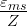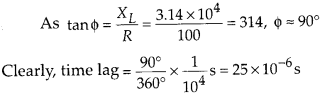Enlightened

# Question 14: NCERT Solutions for 12th Class Physics: Chapter 7-Alternating Current

• 0

Question 14: NCERT Solutions for 12th Class Physics: Chapter 7-Alternating Current

Obtain the answers (a) and (b) in Q. 13, if the circuit is connected to a high frequency supply (240 V, 10 kHz). Hence, explain the statement that at very high frequency, an inductor in a circuit nearly amounts to an open circuit. How does an inductor behave in a dc circuit after the steady state?

Share

1. Solution:
At very high frequency, XL increases to infinitely large, hence circuit behaves as open circuit.(a) Current in the coil, lrms =This current is extremely small. Thus, at high frequencies, the inductive reactance of an inductor is so large that it behaves as an open circuit.

(b)In dc circuit (after steady state), v = 0 and as such XL = 0. In this case, the inductor behaves like a pure resistor as it has no inductive reactance.

Check the complete chapter with solutions.

NCERT Solutions for 12th Class Physics: Chapter 7-Alternating Current

• 0